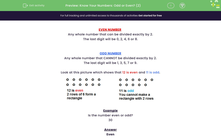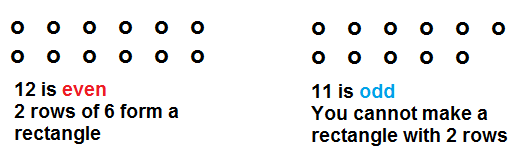# Identify Odd and Even Numbers

In this worksheet, students will work out whether a number is odd or even.Key stage:  KS 1

Curriculum topic:   Number: Multiplication and Division

Curriculum subtopic:   Use Multiplication/Division Facts (2, 5, 10)

Difficulty level:#### Worksheet Overview

In this activity, we are going to be looking at odd and even numbers.

EVEN NUMBERS

Any whole number that can be divided exactly by 2.
The last digit will be 0, 2, 4, 6 or 8.

All these are even numbers: 4, 16, 32, 400, 1258

ODD NUMBERS

Any whole number that cannot be divided exactly by 2.
The last digit will be 1, 3, 5, 7 or 9.

All these are odd numbers: 7, 15, 53, 69, 403, 2671

Look at this picture which shows that 12 is even and 11 is odd.Example

Is this number even or odd?

13

Odd

It can't be divided by 2 exactly.

Also, the last digit is 3.

Have you got all that stored in your memory?Let's move on to some questions.

### What is EdPlace?

We're your National Curriculum aligned online education content provider helping each child succeed in English, maths and science from year 1 to GCSE. With an EdPlace account you’ll be able to track and measure progress, helping each child achieve their best. We build confidence and attainment by personalising each child’s learning at a level that suits them.

Get started